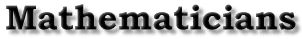| The Abstractor | Elementary Notions | A Fourth Dimension | A Division Algebra | Closure | An Example of Hamilton's Work | Bibliography | Back to the front page Elementary Notions Joseph Johnson Hamilton began his attempt to beautify algebra around 1830. By 1843, his task was completed, though his goal was not fully realized. During this decade or so, he argued that a lower-level definition of algebra was more applicable to the ``real" world. He even aligned himself against the seemingly abstract laws that De Morgan had put forth in 1841. Of course Hamilton's abstract approach characterizes our modern algebra. For example, De Morgan posited that commutativity was essential in any algebra. But general function composition (including matrix multiplication), one of the most important notions in current mathematics, is non-commutative. In this quest to buttress algebra with better foundations, Hamilton set out to discover a three-dimensional complex number system in the vein of number triplets. Because the world is three-dimensional, he reasoned, the undiscovered system may be a model for our universe. Definitions of equality and addition were easy: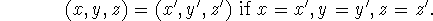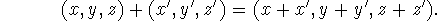Multiplication, on the other hand, was difficult. Realizing that the employment of ``imaginary" units was more practical computationally, Hamilton was willing to concentrate on the non-coordinate version of his idea. The use of i for (0,1,0) and j for (0,0,1) facilitated the attempt at multiplication definition. (Note: In Hamilton's subsequent formal treatise, Elements of Quaternions, he preferred to demonstrate his system in a third manner, namely in vectors and versors. According to Hamilton, a vector was a classical directed line segment. These vectors could be added, subtracted, multiplied, and divided (rotated) according to his definitions. Their lengths could be taken as well. If two vectors were of equal length were divided, the result was a special vector known as a versor. He proceded to note that there exists three very special versors (which we will come to shortly). These versors were designed to be mutually orthogonal, in the directions of the ``rectangular unit-lines" OI, OJ, and OK from our natural world. See back page.) In pondering the definition of his new multiplication, Hamilton first considered how to define ii and jj. He was working under the assumption that the undiscovered structure would be reducible to the ordinary complex number system. That is, the new algebra should be a superset of the already established C. Hence both x+yi+0j and x+0i+zj (two-dimensional elements from the new algebra) should behave as they did in the old algebra. That is, he forced the following equations to be true: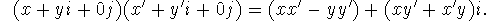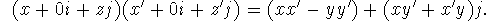Whence, ii=-1 and jj=-1. With this, he had a notion of how to multiply the triplets whose coefficients were not restricted to zero.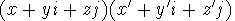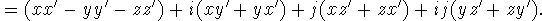How was Hamilton to define ij (ji)? He initially assumed commutativity for his system, which would imply ij=ji; however, the following computation convinced him that commutativity would have to be abandoned.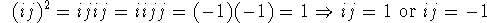Thus, if the undiscovered system was to be commutative, ij would have to be 1 or -1. Either case was unacceptable to Hamilton, because he was working under a special assumption about the lengths of his vectors. His system was supposed to be natural, so he took the length (modulus) of a general vector x+yi+zj to be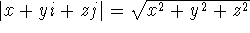(in accordance with the Pythagorean Theorem). Upon this distance function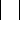he placed the following restriction: The product of the lengths of two vectors must equal the length of the product of the two vectors. Hamilton called this: ``The rule of the Modulus." If he had chosen ij to be in fact any real number, what he called ``the Rule" would be violated. Hamilton also had the intuition to see that his length function would be crucial for division to be possible (see below). Thus if Hamilton's system was to have the requisite modulus function (and possibly division), ij could be neither 1 nor -1, and commutativity would have to be abandoned. | The Abstractor | Elementary Notions | A Fourth Dimension | A Division Algebra | Closure | An Example of Hamilton's Work | Bibliography | Back to the front page